﻿ 这个问题，计算机就算想破了脑袋也解决不了 - 18克# 这个问题，计算机就算想破了脑袋也解决不了

249℃IBM 研究实验室的机器学习专家 Udi Aharoni 根据图灵的证明方法制作了清晰易懂的动画，我们一起来看看吧。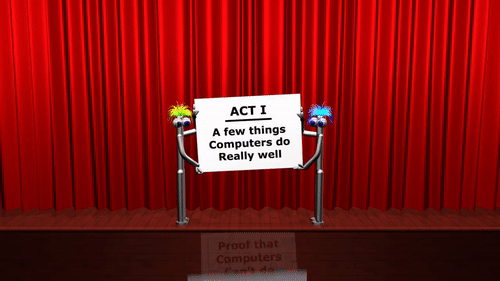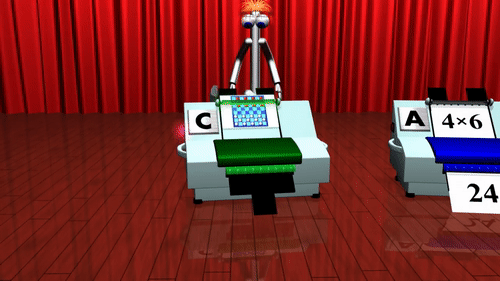emmm，其实，人类已经知道有一类问题，计算机永远也算不出答案。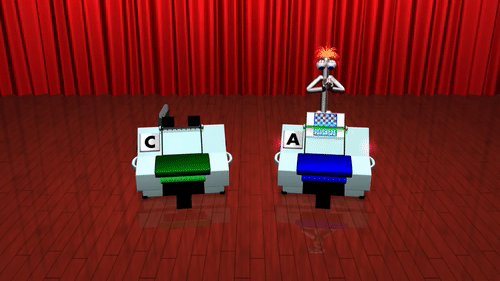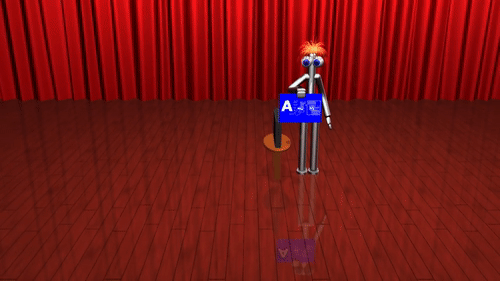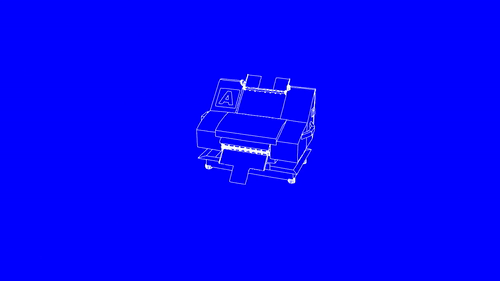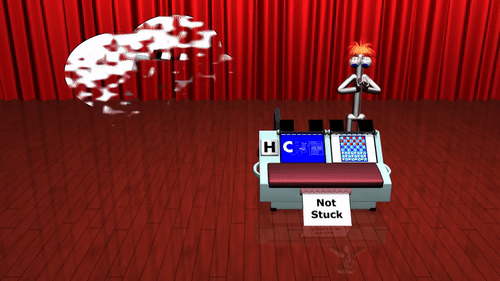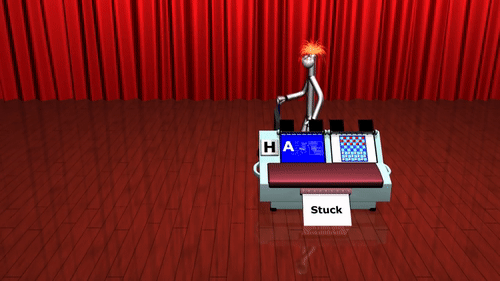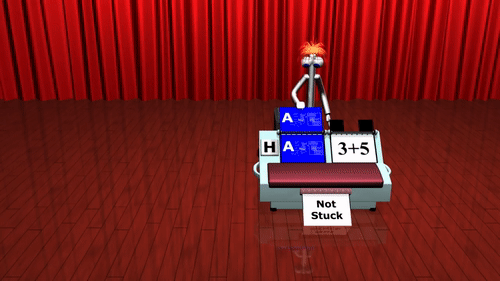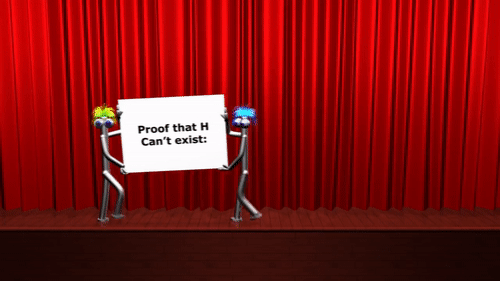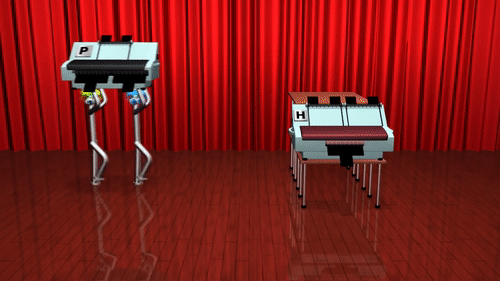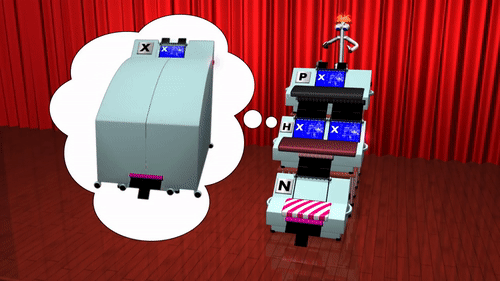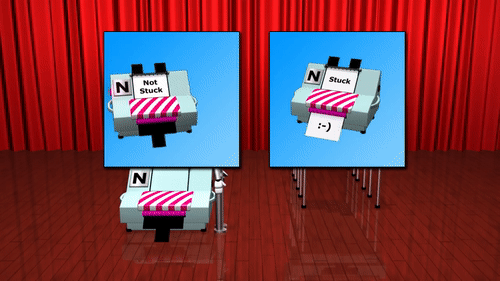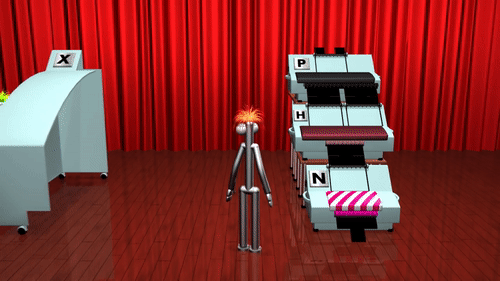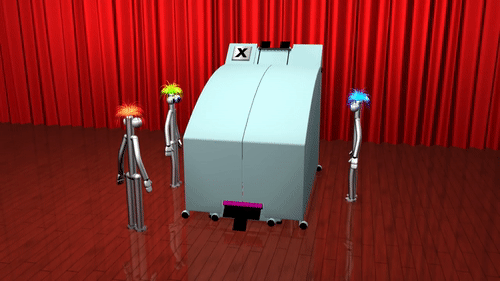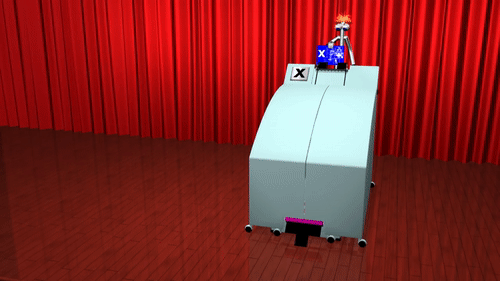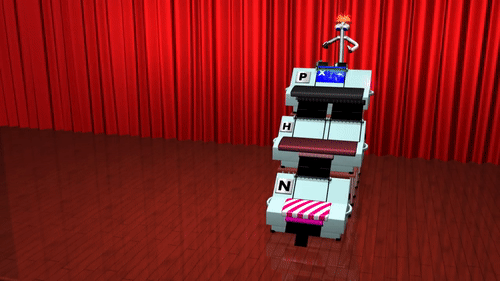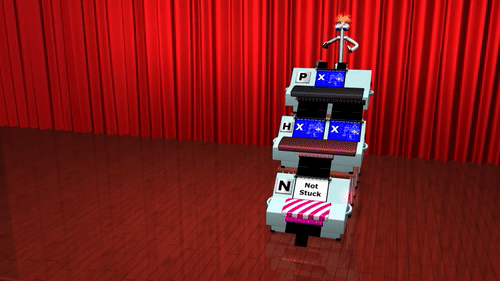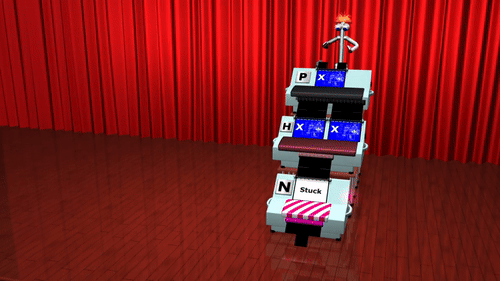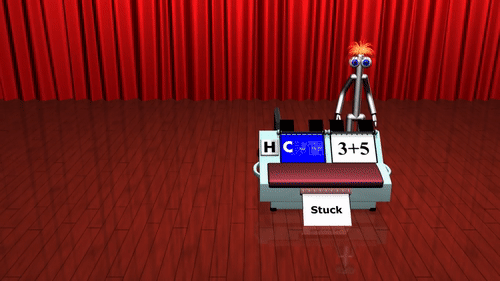4 = 2 + 2

6 = 3 + 3

8 = 3 + 5

10 = 3 + 7 = 5 + 5

12 = 5 + 7

14 = 3 + 11 = 7 + 7*文章为作者独立观点，不代表虎嗅网立场